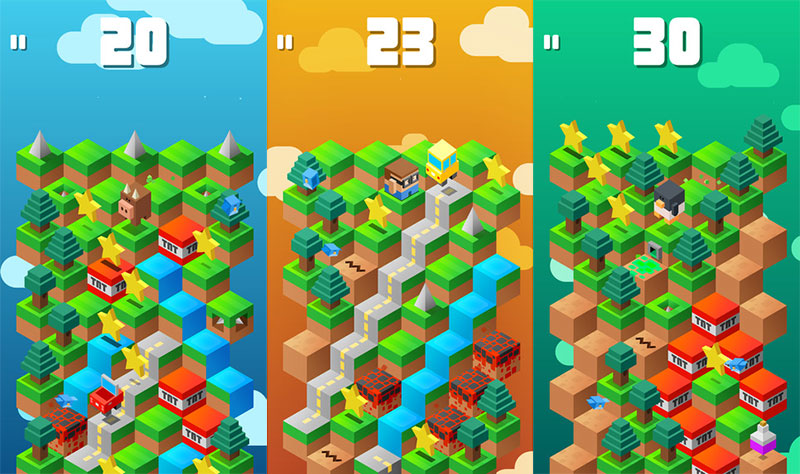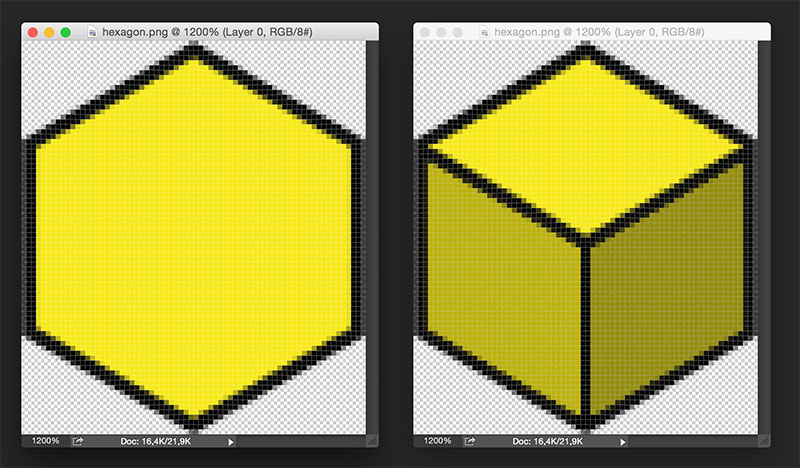# The hexagonal concept behind iOS blockbuster Down The Mountain

Are you playing Umbrella GamesDown The Mountain? I already showed you how to create the prototype of another game made by Umbrella guys, the funny Boom Dots, and this is the time to have a quick look at their new blockbuster, Down The Mountain.Down The Mountain is a unique and fast-paced arcade game that will keep you hooked for hours. The goal of the game is to descend from an infinite mountain, while avoiding many obstacles and enemies, and grabbing stars and power-ups. The game is an endless runner where isometric cubes simulate a 3D mountain. Probably the very first game to introduce thus feature was Q*bert back in 1982. Let’s have a look at the isometric cubes, and you will notice they are just 2D hexagons painted to look like 3D cubes. Let’s have a look at the hexagon image used in he post how to find adjacent tiles in hexagonal maps – ALL and EVERY case explained, and let’s draw a couple of lines and paint a couple of shades:… and here is the isometric cube. So we can modify the script published in the post how to find adjacent tiles in hexagonal maps – ALL and EVERY case explained – actually we are just removing some lines – to create the environment where the game will take place: Move the mouse around the stage and you will see current cube highlighted in green and possible moves highlighted in red. Here is the source code:
```window.onload = function() {

var game = new Phaser.Game(480, 480, Phaser.CANVAS, "", {preload: onPreload, create: onCreate});

var hexagonWidth = 70;
var hexagonHeight = 80;
var gridSizeX = 9;
var gridSizeY = 7;
var columns = [Math.ceil(gridSizeX/2),Math.floor(gridSizeX/2)];
var moveIndex;
var sectorWidth = hexagonWidth;
var sectorHeight = hexagonHeight/4*3;
var marker;
var hexagonGroup;
var hexagonArray = [];

}

function onCreate() {
game.stage.backgroundColor = "#ffffff"
for(var i = 0; i < gridSizeY/2; i ++){
hexagonArray[i] = [];
for(var j = 0; j < gridSizeX; j ++){
if(gridSizeY%2==0 || i+1<gridSizeY/2 || j%2==0){
var hexagonX = hexagonWidth*j/2;
var hexagonY = hexagonHeight*i*1.5+(hexagonHeight/4*3)*(j%2);
hexagonArray[i][j]=hexagon;
hexagonText.font = "arial";
hexagonText.fontSize = 12;
}
}
}
hexagonGroup.x = (game.width-hexagonWidth*Math.ceil(gridSizeX/2))/2;
if(gridSizeX%2==0){
hexagonGroup.x-=hexagonWidth/4;
}
hexagonGroup.y = (game.height-Math.ceil(gridSizeY/2)*hexagonHeight-Math.floor(gridSizeY/2)*hexagonHeight/2)/2;
if(gridSizeY%2==0){
hexagonGroup.y-=hexagonHeight/8;
}
marker.anchor.setTo(0.5);
marker.visible=false;
}

function checkHex(){
var candidateX = Math.floor((game.input.worldX-hexagonGroup.x)/sectorWidth);
var candidateY = Math.floor((game.input.worldY-hexagonGroup.y)/sectorHeight);
var deltaX = (game.input.worldX-hexagonGroup.x)%sectorWidth;
var deltaY = (game.input.worldY-hexagonGroup.y)%sectorHeight;
if(candidateY%2==0){
candidateX--;
candidateY--;
}
candidateY--;
}
}
else{
if(deltaX&gt;=hexagonWidth/2){
candidateY--;
}
}
else{
candidateY--;
}
else{
candidateX--;
}
}
}
placeMarker(candidateX,candidateY);
}

function placeMarker(posX,posY){
for(var i = 0; i < gridSizeY/2; i ++){
for(var j = 0; j < gridSizeX; j ++){
if(gridSizeY%2==0 || i+1<gridSizeY/2 || j%2==0){
hexagonArray[i][j].tint = 0xffffff;
}
}
}
if(posX<0 || posY<0 || posY&gt;=gridSizeY || posX&gt;columns[posY%2]-1){
marker.visible=false;
}
else{
marker.visible=true;
marker.x = hexagonWidth*posX;
marker.y = hexagonHeight/4*3*posY+hexagonHeight/2;
if(posY%2==0){
marker.x += hexagonWidth/2;
}
else{
marker.x += hexagonWidth;
}
var markerX = posX*2+posY%2;
var markerY = Math.floor(posY/2);
hexagonArray[markerY][markerX].tint = 0x00ff00;
if(markerY+markerX%2<gridSizeY/2 &amp;&amp; (gridSizeY%2==0 || markerY<Math.floor(gridSizeY/2))){
// left
if(markerX-1&gt;=0){
hexagonArray[markerY+markerX%2][markerX-1].tint = 0xff0000;
}
// right
if(markerX+1<gridSizeX){
hexagonArray[markerY+markerX%2][markerX+1].tint = 0xff0000;
}
}
}
}
}
```
Just modifying an old post we were able to create the environment needed to run Down The Mountain game. Next time we will build the game prototype itself, meanwhile download the source code.
214 GAME PROTOTYPES EXPLAINED WITH SOURCE CODE
// 1+2=3
// 10000000
// 2 Cars
// 2048
// Avoider
// Ballz
// Block it
// Blockage
// Bloons
// Boids
// Bombuzal
// Breakout
// Bricks
// Columns
// CubesOut
// Dots
// DROP'd
// Dudeski
// Eskiv
// Filler
// Fling
// Globe
// HookPod
// Hundreds
// InkTd
// Iromeku
// Lumines
// Magick
// MagOrMin
// Maze
// Memdot
// Nano War
// Nodes
// o:anquan
// Ononmin
// Pacco
// Phyballs
// Platform
// Poker
// Pool
// Poux
// Pudi
// qomp
// Racing
// Renju
// SameGame
// Security
// Sling
// Slingy
// Sokoban
// Splitter
// Sproing
// Stack
// Stringy
// Sudoku
// Tetris
// Threes
// Toony
// Turn
// TwinSpin
// vvvvvv
// Wordle
// Worms
// Yanga
// Zhed
// zNumbers